Superconductivity

This free course is available to start right now. Review the full course description and key learning outcomes and create an account and enrol if you want a free statement of participation.

Free course

3.2 Magnetic field in a perfect conductor

When discussing the Meissner effect in Subsection 2.3, we argued qualitatively that a material that just had the property of zero resistance – a perfect conductor rather than a superconductor – would maintain a constant magnetic field in its interior, and would not expel any field that was present when the material became superconducting. We shall now show how that conclusion follows from an application of Maxwell's equations to a perfect conductor. We can then see what additional assumptions are needed to account for the Meissner effect in a superconductor.

We assume that the electrons in a perfect conductor (or a proportion of them) are not scattered, and therefore the current density is governed by Equation 3. However, we shall use the subscript ‘pc’ (for perfect conductor) here to indicate that we are not dealing with a superconductor. We are interested in the magnetic field in a perfect conductor, so we shall apply Maxwell's equations to this situation. Faraday's law is valid in all situations,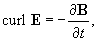and if we substitute for E using Equation 3, we obtain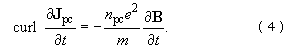Looking now at the Ampère-Maxwell law, curl H = J﻿f + ∂﻿D/∂﻿t, we shall assume that our perfect conductor is either weakly diamagnetic or weakly paramagnetic, so that

μ1 and HB/μ0 are very good approximations.

We shall also omit the Maxwell term, ∂﻿D/∂﻿t, since this is negligible for the static, or slowly-varying, fields that we shall be considering. With these approximations, the Ampère-Maxwell law simplifies to Ampère's law,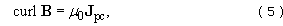where use of the subscript pc for the current density reminds us that the free current J﻿f is carried by the perfectly-conducting electrons. We now use this expression to eliminate J﻿pc from Equation 4: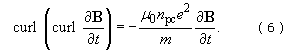We can use a standard vector identity from inside the back cover to rewrite the left-hand side of this equation: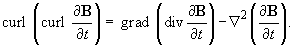The no-monopole law, div B = 0, means that the first term on the right-hand side of this equation is zero, so Equation 6 can be rewritten as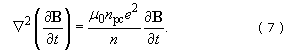This equation determines how ∂﻿B﻿/﻿∂﻿t varies in a perfect conductor.

We shall look for the solution to Equation 7 for the simple geometry shown in Figure 14; a conductor has a boundary corresponding to the plane z = 0, and occupies the region z > 0, with a uniform field outside the conductor given by B﻿0 = B﻿0﻿e﻿x.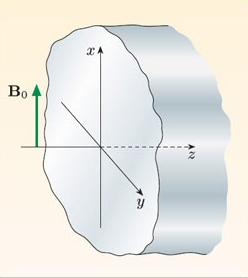Figure 14 A plane boundary between a conductor and air.

The uniform external field in the x-direction means that the field inside the conductor will also be in the x-direction, and its strength will depend only on z. So Equation 7 reduces to the one-dimensional form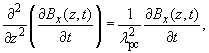where we have simplified the equation, for reasons that will soon become clear, by writing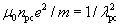The general solution of this equation is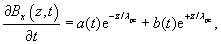where a and b are independent of position. The second term on the right-hand side corresponds to a rate of change of field strength that continues to increase exponentially with distance from the boundary; since this is unphysical, we set b = 0. The boundary condition for the field parallel to the boundary is that H is continuous, and since we are assuming that μ1 in both the air and the conductor, this is equivalent to B﻿ being the same on either side of the boundary at all times. This means that ∂﻿B/∂﻿t is the same on either side of the boundary, so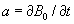and the field within the perfect conductor satisfies the equation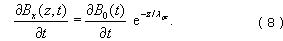This indicates that any changes in the external magnetic field are attenuated exponentially with distance below the surface of the perfect conductor. If the distance λpc is very small, then the field will not change within the bulk of the perfect conductor, and this is the behaviour that we described qualitatively in Subsection 2.3. Note that this does not mean the magnetic field must be expelled: flux expulsion requires B = 0, rather than just ∂﻿B/∂﻿t = 0. So how do we modify the description that we have given of a perfect conductor so that it describes a superconductor and leads to a prediction that B = 0?

SMT359_1